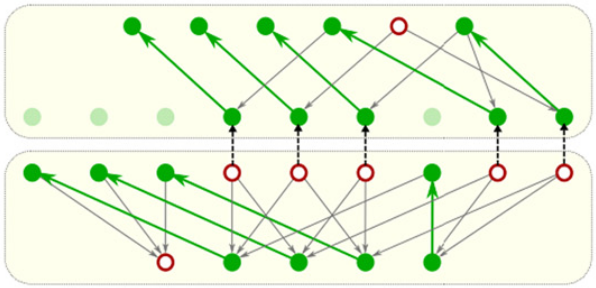#### Approximation algorithms for Max Morse matching.

Abhishek Rathod, Talha Bin Masood and Vijay Natarajan.
Computational Geometry: Theory and Applications, 61, 2017, 1-23.
[Elsevier link]

#### Abstract

In this paper, we prove that the Max Morse Matching Problem is approximable, thus resolving an open problem posed by Joswig and Pfetsch. For D-dimensional simplicial complexes, we obtain a (D+1)/(D2+D+1)-factor approximation ratio using a simple edge reorientation algorithm that removes cycles. For D≥5, we describe a 2/D-factor approximation algorithm for simplicial manifolds by processing the simplices in increasing order of dimension. This algorithm leads to 1/2-factor approximation for 3-manifolds and 4/9-factor approximation for 4-manifolds. This algorithm may also be applied to non-manifolds resulting in a 1/(D+1)-factor approximation ratio. One application of these algorithms is towards efficient homology computation of simplicial complexes. Experiments using a prototype implementation on several datasets indicate that the algorithm computes near optimal results.

[PDF]